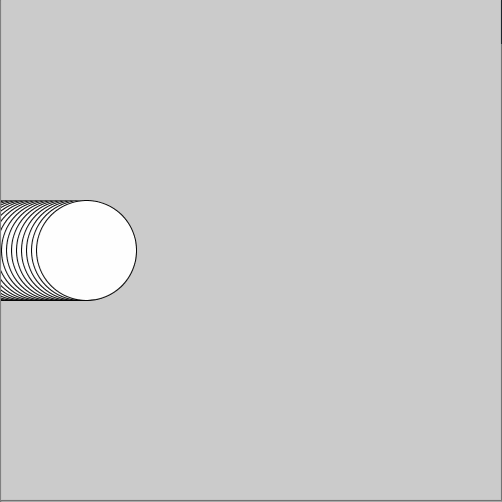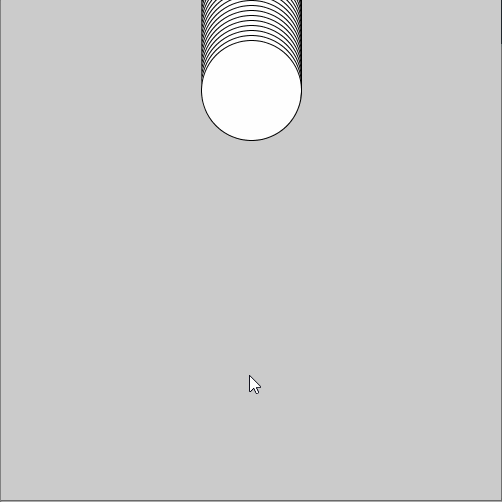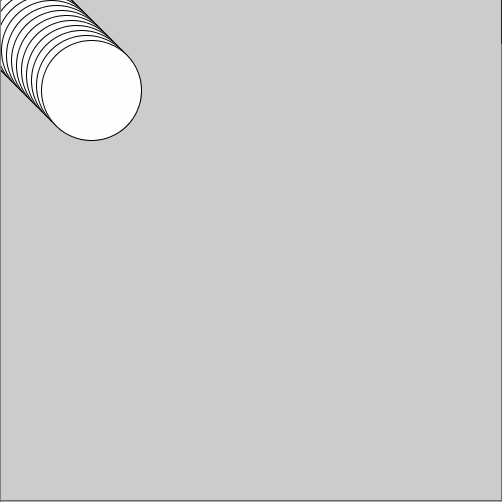# What If?

## What If It Was an UFO

Do this

Copy the program completed in the video from below and test it.

``````int ufoX = 0;

void setup(){
size(500, 500);
fill(250, 250, 0);
}

void draw(){
ellipse(ufoX, 250, 200, 80);
ufoX = ufoX + 1;
if(ufoX > 500){
ufoX = 0;
}
}
``````

Change the program so that the ellipse moves completely over the right side of the canvas before appearing again from the left.

HINT: You have to edit the condition of the if-structure. Try different threshold-values. Below there are two examples.

`ballX > 200` `ballX > 550`

The result should look like the one in the animation below.

What is the right condition for the UFO moving completely over the right side of the canvas before appearing again from the left?

Educator notes

In the task, students should edit the program so that the UFO goes totally past the right edge before appearing again from the left. The correct code is given below.

``````int ufoX = 0;

void setup(){
size(500, 500);
}

void draw(){
ellipse(ufoX, 250, 200, 80);
ufoX = ufoX + 1;
if(ufoX > 600){   // the test-part
ufoX = 0;       // the statement-part
}
}
``````

The condition has to be `ballx > 600`, because the width of the UFO is 200. When the x-coordinate of the center of the UFO is 600, the left end of the UFO is in the point 500 which is the right end of the canvas.

To produce the exact result in the animation, students have to edit the statement-part too. It has to be `ballX = -100` because when the x-coordinate of the center is in that point, the x-coordinate of the right end of the UFO is 0. We haven’t used negative coordinate-points before so this part might be challenging to figure out. This can be a nice extra-challenge for the more advanced students!

## Bouncing Balls

Do this

Copy the code completed in the video (below).

``````int ballX = 0;
int speed = 5;

void setup(){
size(500, 500);
}

void draw(){
ellipse(ballX, 250, 100, 100);
ballX = ballX + speed;
if(ballX > 500 || ballX < 0){
speed = speed * -1;
}
}
``````

Edit the code to produce the result animated below in the center. Try to produce the result animated on the right. You need to combine the two earlier versions! If it feels difficult, you can find help below!``````if(ballX &#62; 500 &#124;&#124; ballX &#60; 0){
speed = speed * -1;
}
``````
What does the expression above do?

Educator notes

Multiplying with negative number always changes a positive number to negative and vice versa. It’s a handy trick when you want to change the direction of a moving object.

The || -sign means OR, as explained on the video.

Activity: if-structures can be easier to understand if you read them out loud, like: “if ballX is greater than 500 or if it is less than zero, change the sign of the speed so that the ball bounces back from the edge”. This is a way to form an analogy between everyday language and often technical programming jargon.

## There and Back Again

Do this

Copy the code completed in the video from below and test it.

Change the starting point of the ball by changing the initial values of ballX and ballY.

Change the horizontal and vertical speeds of the ball by changing the initial values of speedX and speedY.

``````int ballX = 0;
int ballY = 150;
int speedX = 5;
int speedY = 3;

void setup(){
size(500, 500);
}

void draw(){
ellipse(ballX, ballY, 100, 100);
ballX = ballX + speedX;
ballY = ballY + speedY;
if(ballX > 500 || ballX < 0){
speedX = speedX * -1;
}
if(ballY > 500 || ballY < 0){
speedY = speedY * -1;
}
}
``````

Increase the initial value of ballX. What happens?
Increase the initial value of ballY. What happens?
Increase the value of speedY. What happens?

Educator notes

When the ball doesn’t start from the top-left corner, one of the coordinates “has a head start”. For example on the video, ballX is 0 and ballY is 150 in the start. So when ballY becomes 500, ballX is still just 350 and keeps increasing. That’s why the ball bounces back in the vertical direction before bouncing back in the horizontal direction.

In mathematics, the steepness of a line is called a slope or* a gradient*. For example a slope of 1 means that the x- and y-coordinates increase at the same rate. A slope of 2 means that the the line is less steep: when x-coordinate increases by two, y-coordinate increases just by one.

You can calculate the slope of the ball by dividing speedX by speedY. E.g. if speedX is 2 and speedY is 5, the slope is 2/5 = 0,4.

## Refreshing the Background

Do this

Add a fill-command and a rect-command to the start of the draw-method like in the video. You can choose your own filling color.

``````fill(0);
rect(0, 0, 500, 500);
``````

Add a second parameter to the fill-command. Experiment with different values and see how it affects the result.

``````fill(0, 10);
rect(0, 0, 500, 500);
``````

If you use two parameters in the fill-command, what does the first parameter do?
If you use two parameters in the fill-command, what does the second parameter do?

Educator notes

It's possible to refresh the background with the background-command too. But while using the background-command, it's not possible to change the opacity of the color. That's why a canvas-size rectangle is sometimes a more handy solution.

Opacity was introduced already in the Autumn Vibes-example but it may still require a little bit extra explaining.

• If you use four parameters, the first three define the color (RGB) and the fourth is the opacity.
• If you use just two parameters, the first is the grayscale color and the second one is the opacity. All the parameters inside stroke and fill -commands have to be between 0 and 255.

If you use opacity of 0, the're is no color (opacity is 0 %). The value 127 means that the opacity is 50 % (255/2 -> 127). You can use the stroke- and fill -commands with many parameter-combinations, check the reference for more information.

In the next chapter, students learn to set an image to the background of a sketch. If someone wants to do it now already, here's the info-page in Processing reference.